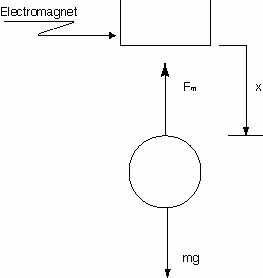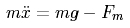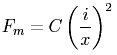2.003 | Spring 2005 | Undergraduate

# Modeling Dynamics and Control I

Study Materials

## System Model

Main | Hardware | System Model | Linearized Control | Nonlinear Control | Movies | Technical Details | Credits

The first step in controlling a system is deriving an accurate model for the system. Each of the system elements’ behaviors can be derived from basic physics. In the examples below, many of the equations are left in terms of constants. These constants are dependent on materials and geometry, and are thus specific to the hardware. Procedures for measuring these constants are provided in Yi Xie’s MEng thesis (see the technical details section).Free Body Diagram of the Plant. (Fig. M1)

As shown in the free body diagram above, two forces act on the steel ball: gravity and the electro-magnetic force from the coils. The equation of motion for the ball is therefore:Eq. M1.

In the simplest model, Fm, the electromagnetic force, is proportional to the square of the current passing through the inductor and inversely proportional to the square of the distance between the magnet and the steel ball. The constant C is a function of the physical construction of the electromagnet.Eq. M2.

The power amplifier is set up as an actively controlled current source, so we assume current to be the controlled variable. This means that we can ignore coil inductance and speed voltage effects. Combining the two equations given above gives:Eq. M3.

## Course Info

Spring 2005
##### Learning Resource Types
Demonstration Videos
Exams
Problem Sets